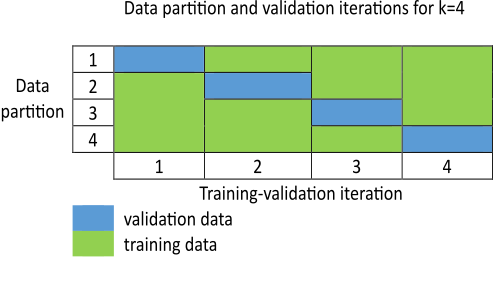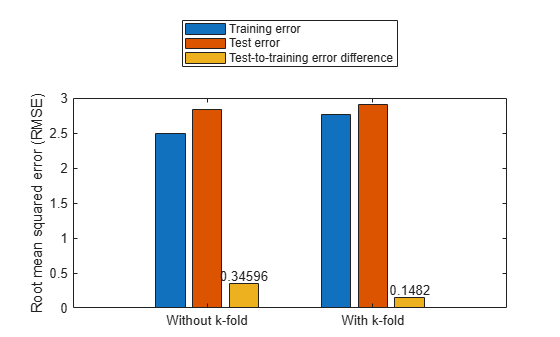# FIS Parameter Optimization with K-fold Cross Validation

This example shows parameter optimization of a fuzzy inference system (FIS) using k-fold cross validation. This example uses genetic algorithm (GA) optimization, which requires Global Optimization Toolbox™ software.

### Data Overfitting in FIS Parameter Tuning

Data overfitting is a common problem in FIS parameter optimization. When overfitting occurs, the tuned FIS produces optimized results for the training data set but performs poorly for a test data set. Due to overtuning, the optimized FIS parameter values pick up noise from the training data set and lose the ability to generalize to new data sets. The difference between the training and test performance increases with the increased bias of the training data set.

To overcome the data overfitting problem, a tuning process can stop early based on an unbiased evaluation of the model using a separate validation dataset. Such a validation dataset can also increase bias if it does not accurately represent the problem space. To overcome bias from the validation data set, a k-fold cross-validation approach is commonly used. Here, the training data is randomly shuffled and then divided into $\mathit{k}$ partitions, as shown in the following figure. For each training-validation iteration, a different partition is used for validation, with the remaining data used for testing. Therefore, each data partition is used once for validation and $\mathit{k}-1$ times for training.Each training-validation iteration runs for $\mathit{n}$ cycles; however, an iteration can stop early at ${\mathit{n}}_{\mathit{k}}<\mathit{n}$ and advance to the next iteration if an increase in the validation cost exceeds a predefined threshold value. The optimized model at the end of the ${\mathit{k}}^{\mathrm{th}}$ iteration is used as the output of the k-fold cross-validation process.

This example shows how using k-fold cross validation with the `tunefis` function prevents data overfitting compared to parameter tuning that does not use k-fold cross validation.

### Tune FIS without K-Fold Validation

This example describes a data overfitting problem for automobile fuel consumption prediction. It uses several automobile profile attributes to predict fuel consumption. The training data is available in the University of California at Irvine Machine Learning Repository and contains data collected from automobiles of various makes and models.

This example uses the following six input data attributes to predict the output data attribute MPG with a FIS:

1. Number of cylinders

2. Displacement

3. Horsepower

4. Weight

5. Acceleration

6. Model year

Load the data using the `loaddata` utility function shown at the end of the example. This function creates training and test data sets.

`[data,varName,trnX,trnY,testX,testY] = loadData;`

Create an initial FIS based on the input and output data attributes using the `constructFIS` utility function.

`fisin = constructFIS(data,varName);`

Create an option set for tuning the FIS. The default option set uses GA for optimization.

`options = tunefisOptions;`

If you have Parallel Computing Toolbox™ software, you can improve the speed of the tuning process by setting `options.UseParallel` to `true`. If you do not have Parallel Computing Toolbox software, set `options.UseParallel` to `false`.

To demonstrate the data overfitting problem, this example uses a maximum of 100 generations to tune the rules.

`options.MethodOptions.MaxGenerations = 100;`

Tune the FIS using the specified tuning data and options. Tuning rules using the `tunefis` function takes several minutes. This example uses a flag, `runtunefis`, to either run the `tunefis` function or load pretrained results. To load the pretrained results, set `runtunefis` to `false`.

`runtunefis = false;`

To demonstrate the data overfitting problem, use the following performance measures:

• Training error — Root-mean-square error (RMSE) between the expected training output and the actual training output obtained from the tuned FIS.

• Test error — RMSE between the expected test output and the actual test output obtained from the tuned FIS.

• Function count — The total number of evaluations of the cost function for tuning the FIS.

In this example, use only rule parameter settings for tuning the FIS.

Since GA optimization uses random search, to obtain reproducible results, initialize the random number generator to its default configuration.

```if runtunefis % Get rule parameter settings. [~,~,rule] = getTunableSettings(fisin); % Set default random number generator. rng('default') % Tune rule parameters. [outputFIS,optimData] = tunefis(fisin,rule,trnX,trnY,options); % Get the trainig error. trnErrNoKFold = optimData.tuningOutputs.fval % Calculate the test error. evalOptions = evalfisOptions("EmptyOutputFuzzySetMessage","none", ... "NoRuleFiredMessage","none","OutOfRangeInputValueMessage","none"); actY = evalfis(outputFIS,testX,evalOptions); del = actY - testY; testErrNoKFold = sqrt(mean(del.^2)) % Get the function count. fcnCountNoKFold = optimData.totalFcnCount save tuningWithoutKFoldValidation trnErrNoKFold testErrNoKFold fcnCountNoKFold else % Load the pretrained results. results = load('tuningWithoutKFoldValidation.mat'); trnErrNoKFold = results.trnErrNoKFold testErrNoKFold = results.testErrNoKFold fcnCountNoKFold = results.fcnCountNoKFold end```
```trnErrNoKFold = 2.4952 ```
```testErrNoKFold = 2.8412 ```
```fcnCountNoKFold = 19210 ```

The higher value of the test error as compared to the training error indicates that the trained FIS is more biased to the training data.

### Tune FIS Parameters with K-Fold Validation

You can use k-fold cross validation in FIS parameter optimization by setting `options.KFoldValue` to a value greater than or equal to 2. For this example, set the k-fold value to 4.

`options.KFoldValue = 4;`

To specify a tolerance value for which stop a k-fold tuning process, set the `options.ValidationTolerance` property. For this example, set the tolerance value to 0.02. This tolerance value configures the k-fold tuning process to stop if the current validation cost increases by more than 2% of the minimum validation cost found up to that point in the tuning process.

`options.ValidationTolerance = 0.02;`

For a noisy data set, you can compute a moving average of the validation cost by setting the `options.ValidationWindowSize` property to a value greater than 1. For this example, set the validation window size to 2.

`options.ValidationWindowSize = 2;`

Restrict the maximum number of generations in each tuning process to 25 so that the total number of generations in the 4-fold tuning process is the same as the previous case.

`options.MethodOptions.MaxGenerations = 25;`

Tune the FIS with k-fold validation.

```if runtunefis % Set default random number generator. rng('default') % Tune the FIS. [outputFIS,optimData] = tunefis(fisin,rule,trnX,trnY,options); % Get the trainig error. trnErrWithKFold = optimData.tuningOutputs(end).fval % Calculate the test error. actY = evalfis(outputFIS,testX,evalOptions); del = actY - testY; testErrWithKFold = sqrt(mean(del.^2)) % Get the function count. fcnCountWithKFold = optimData.totalFcnCount save tuningWithKFoldValidation trnErrWithKFold testErrWithKFold fcnCountWithKFold else % Load the pretrained results. results = load('tuningWithKFoldValidation.mat'); trnErrWithKFold = results.trnErrWithKFold testErrWithKFold = results.testErrWithKFold fcnCountWithKFold = results.fcnCountWithKFold end```
```trnErrWithKFold = 2.7600 ```
```testErrWithKFold = 2.9082 ```
```fcnCountWithKFold = 5590 ```

Plot the test-to-training error differences for training both with and without k-fold cases.

```figure cats = categorical({'Without k-fold','With k-fold'}); cats = reordercats(cats,{'Without k-fold','With k-fold'}); data = [trnErrNoKFold testErrNoKFold testErrNoKFold-trnErrNoKFold; ... trnErrWithKFold testErrWithKFold testErrWithKFold-trnErrWithKFold]; b = bar(cats,data); ylabel('Root mean square error (RMSE)') text(b(3).XEndPoints,b(3).YEndPoints,string(b(3).YData),'HorizontalAlignment','center',... 'VerticalAlignment','bottom') legend('Training error','Test error','Test to training error difference', ... 'Location','northoutside')```The test error performance is similar in both cases. However, the difference in the training and test errors with k-fold validation is less than without k-fold validation. Therefore, the k-fold validation reduces the bias of the training data and produces better generalized FIS parameter values. The total function count during k-fold validation is less than the count without k-fold validation.

```disp(table(fcnCountNoKFold,fcnCountWithKFold, ... 'VariableNames',["Without k-fold" "With k-fold"], ... 'RowName',"Function counts"))```
``` Without k-fold With k-fold ______________ ___________ Function counts 19210 5590 ```

Therefore, k-fold validation reduces the number of generations in each GA optimization cycle, reducing FIS parameter overfitting. The overall k-fold validation results can be further improved by experimenting with different k-fold, tolerance, and window size values.

### Conclusion

With k-fold cross validation, you can achieve better FIS parameter generalization with fewer function counts as compared to a similar tuning process without run-time cross validation.

In general, use the following process for FIS parameter optimization with k-fold validation:

1. Start with a validation tolerance of 0 and a window size of 1, which provide the minimal k-fold performance.

2. Increase the k-fold value to achieve your desired performance. In general, use a k-fold value less than or equal to 10.

3. Increase the tolerance value to achieve your desired performance.

4. Increase the window size to achieve your desired performance.

5. You can repeat steps 3 and 4 in a loop to find optimal validation settings.

Higher values of tolerance, window size, and k-fold value introduce data overfitting in the optimized FIS parameter values. Therefore, use smaller values to achieve your desired tuning performance.

### Local Functions

```function [data,varName,trnX,trnY,testX,testY] = loadData % Load the data. Each row of the dataset obtained from the repository represents % a different automobile profile. Data contains 7 columns, where the first six % columns contain the following input attributes. % - Number of cylinders % - Displacement % - Horsepower % - Weight % - Acceleration % - Model year % The seventh column contains the output attribute, MPG. [data,name] = loadgas; % Remove leading and trailing whitespace from the attribute names. varName = strtrim(string(name)); % Create input and output data sets. n = size(data,2); x = data(:,1:n-1); y = data(:,n); % Create training and test data sets. trnX = x(1:2:end,:); trnY = y(1:2:end,:); testX = x(2:2:end,:); testY = y(2:2:end,:); end function fisin = constructFIS(data,varName) % Create a Sugeno FIS. fisin = sugfis; % Add input and output variables to the FIS, where each variable represents % one of the data attributes. For each variable, use the corresponding % attribute name and range. To reduce the number of rules, use two MFs for % each input variable, which results in 2^6=64 input MF combinations. % Therefore, the FIS uses a maximum of 64 rules corresponding to the input % MF combinations. Both input and output variables use default triangular % MFs, which are uniformly distributed over the variable ranges. dataRange = [min(data)' max(data)']; numINputs = size(data,2)-1; numInputMFs = 2; numOutputMFs = numInputMFs^numINputs; for i = 1:numINputs fisin = addInput(fisin,dataRange(i,:),'Name',varName(i),'NumMFs',numInputMFs); end % To improve data generalization, use 64 MFs for the output variable. % Doing so allows the FIS to use a different output MF for each rule. fisin = addOutput(fisin,dataRange(end,:),'Name',varName(end),'NumMFs',numOutputMFs); fisin.Rules = repmat(fisrule,[1 numOutputMFs]); end```